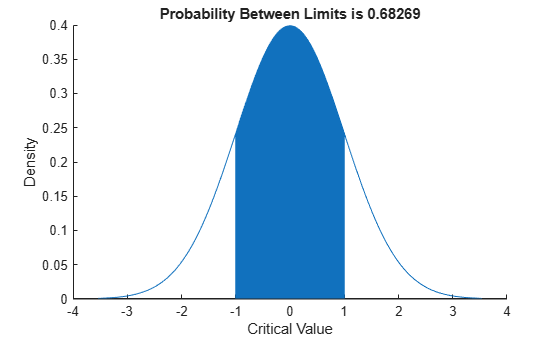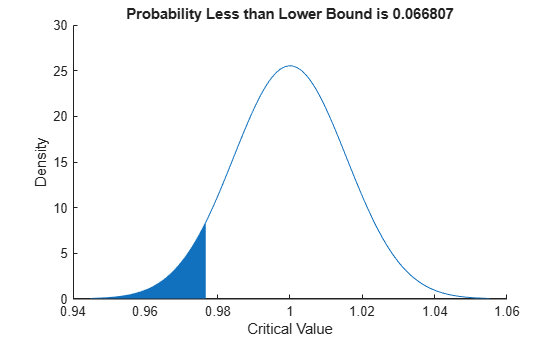# normspec

Normal density plot shading between specifications

## Syntax

``p = normspec(specs)``
``p = normspec(specs,mu,sigma)``
``p = normspec(specs,mu,sigma,region)``
``[p,h] = normspec(___)``

## Description

example

````p = normspec(specs)` plots the standard normal density, shading the portion inside the specification limits given by the two-element vector `specs`, and returns the probability `p` of the shaded area. If `spec` has no lower limit, then set `specs(1)` to `–Inf`; if `spec` has no upper limit, then set `specs(2)` to `Inf`.```
````p = normspec(specs,mu,sigma)` uses a normal density with parameters `mu` and `sigma`.```

example

````p = normspec(specs,mu,sigma,region)` specifies the shading region as either `'inside'` or `'outside'` the specification limits. The default is `'inside'`.```
````[p,h] = normspec(___)` also returns a column vector of chart line objects using any of the input arguments in the previous syntaxes. Use `h` to modify properties of a specific chart line after you create it. For a list of properties, see Line Properties.```

## Examples

collapse all

Create a standard normal density plot, shading the portion inside the specification limits `[-1,1]`.

`p = normspec([-1,1])````p = 0.6827 ```

Create a normal density plot, shading the portion outside the specification limits.

For example, consider a production process that fills cans of paint. The average amount of paint in any can is 1 gallon, but variability in the process produces a standard deviation of 2 ounces (2/128 gallons). Create a normal density plot, shading the portion corresponding to the probability that the cans will be filled under specification by 3 or more ounces.

`p = normspec([1-3/128,Inf],1,2/128,'outside')````p = 0.0668 ```

## Input Arguments

collapse all

Specification limits, specified as a two-element numeric vector. Set `specs(1)` to `–Inf` if there is no lower limit; set `specs(2)` to `Inf` if there is no upper limit.

Data Types: `single` | `double`

Mean of the normal distribution, specified as a scalar value.

Data Types: `single` | `double`

Standard deviation of the normal distribution, specified as a positive scalar value.

Data Types: `single` | `double`

Shading region, specified as either `'inside'` or `'outside'`. The function `normspec` shades the region either `'inside'` or `'outside'` the specification limits.

## Output Arguments

collapse all

Probability of the shaded area, returned as a nonnegative scalar value.

One or more chart line objects, returned as a scalar or vector. These objects are unique identifiers that you use to query and modify properties of a specific chart line. For a list of properties, see Line Properties.